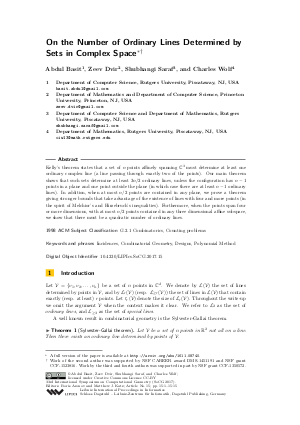Document# On the Number of Ordinary Lines Determined by Sets in Complex Space

### Authors Abdul Basit, Zeev Dvir, Shubhangi Saraf, Charles Wolf## File

LIPIcs.SoCG.2017.15.pdf
• Filesize: 0.51 MB
• 15 pages

## Cite As

Abdul Basit, Zeev Dvir, Shubhangi Saraf, and Charles Wolf. On the Number of Ordinary Lines Determined by Sets in Complex Space. In 33rd International Symposium on Computational Geometry (SoCG 2017). Leibniz International Proceedings in Informatics (LIPIcs), Volume 77, pp. 15:1-15:15, Schloss Dagstuhl - Leibniz-Zentrum für Informatik (2017)
https://doi.org/10.4230/LIPIcs.SoCG.2017.15

## Abstract

Kelly's theorem states that a set of n points affinely spanning C^3 must determine at least one ordinary complex line (a line passing through exactly two of the points). Our main theorem shows that such sets determine at least 3n/2 ordinary lines, unless the configuration has n-1 points in a plane and one point outside the plane (in which case there are at least n-1 ordinary lines). In addition, when at most n/2 points are contained in any plane, we prove a theorem giving stronger bounds that take advantage of the existence of lines with four and more points (in the spirit of Melchior's and Hirzebruch's inequalities). Furthermore, when the points span four or more dimensions, with at most n/2 points contained in any three dimensional affine subspace, we show that there must be a quadratic number of ordinary lines.
##### Keywords
• Incidences
• Combinatorial Geometry
• Designs
• Polynomial Method

## Metrics

• Access Statistics
• Total Accesses (updated on a weekly basis)
0

## References

1. N. Alon. Perturbed identity matrices have high rank: Proof and applications. Combinatorics, Probability and Computing, 18(1-2):3-15, 2009.2. B. Barak, Z. Dvir, A. Wigderson, and A. Yehudayoff. Fractional Sylvester-Gallai theorems. Proceedings of the National Academy of Sciences, 110(48):19213-19219, 2013.3. P. Borwein and W. Moser. A survey of Sylvester’s problem and its generalizations. Aequationes Mathematicae, 40(1):111-135, 1990.4. R. K. Brayton, D. Coppersmith, and A. J. Hoffman. Self-orthogonal latin squares of all orders n ≠ 2, 3, 6. Bulletin of the American Mathematical Society, 80, 1974.5. J. Csima and E. T. Sawyer. There exist 6n/13 ordinary points. Discrete &Computational Geometry, 9(2):187-202, 1993.6. Z. Dvir, S. Saraf, and A. Wigderson. Improved rank bounds for design matrices and a new proof of Kelly’s theorem. In Forum of Mathematics, Sigma, volume 2, page e4. Cambridge University Press, 2014.7. N. Elkies, L. M. Pretorius, and K. Swanepoel. Sylvester-Gallai theorems for complex numbers and quaternions. Discrete &Computational Geometry, 35(3):361-373, 2006.8. T. Gallai. Solution of problem 4065. American Mathematical Monthly, 51:169-171, 1944.9. B. Green and T. Tao. On sets defining few ordinary lines. Discrete &Computational Geometry, 50(2):409-468, 2013.10. A. J. W. Hilton. On double diagonal and cross latin squares. Journal of the London Mathematical Society, 2(4):679-689, 1973.11. F. Hirzebruch. Arrangements of lines and algebraic surfaces. In Arithmetic and geometry, pages 113-140. Springer, 1983.12. L. Kelly. A resolution of the Sylvester-Gallai problem of J.-P. Serre. Discrete &Computational Geometry, 1(1):101-104, 1986.13. L. Kelly and W. Moser. On the number of ordinary lines determined by n points. Canadian Journal of Mathematics, 10:210-219, 1958.14. E. Melchior. Über Vielseite der projektiven Ebene. Deutsche Math, 5:461-475, 1940.15. Th. Motzkin. The lines and planes connecting the points of a finite set. Transactions of the American Mathematical Society, pages 451-464, 1951.16. U. Rothblum and H. Schneider. Scalings of matrices which have prespecified row sums and column sums via optimization. Linear Algebra and its Applications, 114:737-764, 1989.17. J.-P. Serre. Advanced problem 5359. American Mathematical Monthly, 73(1):89, 1966.18. J. J. Sylvester. Mathematical question 11851. Educational Times, 59(98):256, 1893.X

Feedback for Dagstuhl Publishing